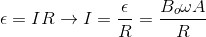# AP Physics C Electricity : Using Faraday's Law

## Example Questions

### Example Question #1 : Using Faraday's Law

A wire loop of areais placed in a magnetic field whose magnitude varies with time according to the equation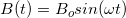. The loop is held in place, perpendicular to the field. If the wire has resistance, what is the magnitude of the maximum instantaneous current induced in the loop?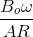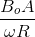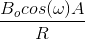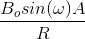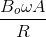Explanation:

Relevant equations: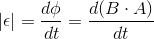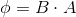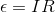Write the expression for the flux using the given expression for the magnetic field strength: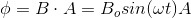Take the derivative of this flux to find the induced emf: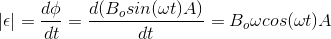Determine the maximum value of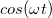, and replace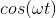with this value, to find the maximum induced emf: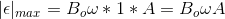Plug this maximum induced emf into, to find the maximum induced current: